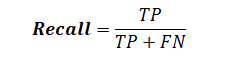# NLP | Partial parsing with Regex

• Last Updated : 23 Feb, 2019
• Defining a grammar to parse 3 phrase types.
• ChunkRule class that looks for an optional determiner followed by one or more nouns is used for noun phrases.
• To add an adjective to the front of a noun chunk, MergeRule class is used.
• Any IN word is simply chunked for the prepositional phrases.
• an optional modal word (such as should) followed by a verb is chunked for the verb phrases.

Code #1 :

 `chunker ``=` `RegexpParser(r``'''``                       ``NP:``                           ``# chunk optional determiner with nouns``                           ``{
?+}``                            ` `                           ``# merge adjective with noun chunk``                           ``{}                            ``                            ` `                       ``PP:``                           ``# chunk preposition``                           ``{} ``                       ``VP:``                           ``# chunk optional modal with verb``                           ``{?} '''``)``     ` `from` `nltk.corpus ``import` `conll2000`` ` `score ``=` `chunker.evaluate(conll2000.chunked_sents())`` ` `print` `(``"Accuracy : "``, score.accuracy())`

Output :

```Accuracy : 0.6148573545757688
```

treebank_chunk corpus is a special version of the treebank corpus and it provides a chunked_sents() method. Duw to its file format, the regular treebank corpus cannot provide that method.

Code #2 : Using treebank_chunk

 `from` `nltk.corpus ``import` `treebank_chunk`` ` `treebank_score ``=` `chunker.evaluate(``        ``treebank_chunk.chunked_sents())`` ` `print` `(``"Accuracy : "``, treebank_score.accuracy()`

Output :

```Accuracy : 0.49033970276008493
```

Chunk Score Metrices
It provides metrics other than accuracy. Of the chunks
Precision means how many were correct.
Recall means how well the chunker did at finding correct chunks compared to how many total chunks there were.Code #3 : Chunk Score Metrices

 `print` `(``"Precision : "``, score.precision())`` ` `print` `(``"\nRecall : "``, score.recall())`` ` `print` `(``"\nLength for missed one : "``, ``len``(score.missed()))`` ` `print` `(``"\nLength for incorrect one : "``, ``len``(score.incorrect()))`` ` `print` `(``"\nLength for correct one : "``, ``len``(score.correct()))`` ` `print` `(``"\nLength for guessed one : "``, ``len``(score.guessed()))`

Output :

```Precision : 0.60201948127375

Recall : 0.606072502505847

Length for missed one : 47161

Length for incorrect one : 47967

Length for correct one : 119720

Length for guessed one : 120526
```

My Personal Notes arrow_drop_up